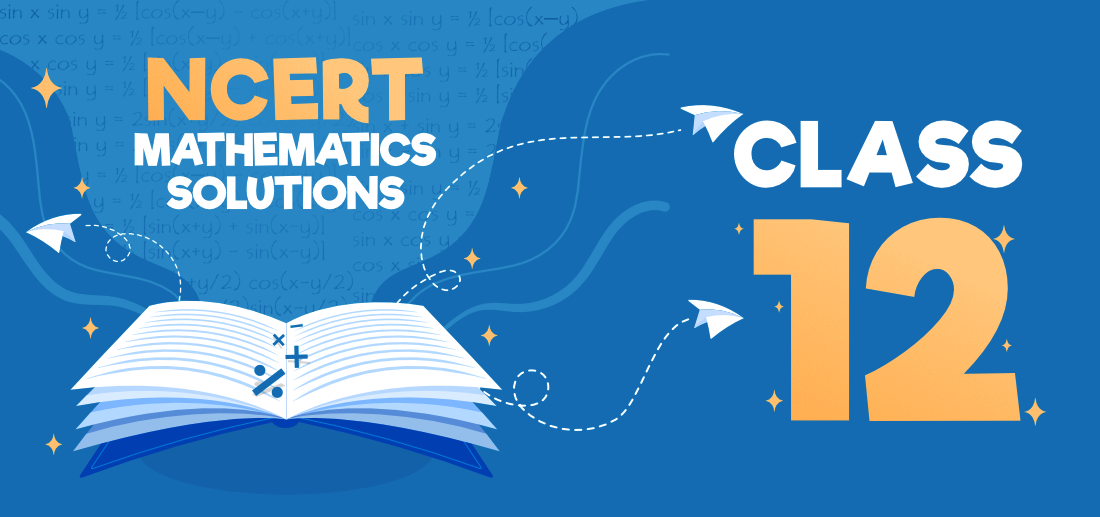NCERT Solutions for Class 12 Maths

• Last Updated : 22 Apr, 2021

NCERT Solutions for Class 12 Maths provides a solid conceptual base for all the topics included in CBSE Class 12 Maths Syllabus. It covers all the important theorems and formulae with detailed explanations to promote a better conceptual understanding in students. The solutions provided by GeeksforGeeks help students to build a deeper understanding of the concepts like Matrices & Determinants, Integrals, Differentials, Etc.Chapter 1: Relations and Functions

The Chapter Relation and functions discuss the introduction of relations and functions, types of relation, types of functions, the composition of functions and invertible function, binary operations, and miscellaneous examples. Here, are total five exercises covered in this chapter. Exercise 1.1 is only based upon the topic types of relations: reflexive, symmetric, transitive, and equivalence relations while the rest of the exercises 1.2, 1.3, and 1.4 are based on one to one and onto functions, composite functions, the inverse of a function. However, miscellaneous exercises cover standard problems based on all the mentioned topics of this chapter.

Chapter 2: Inverse Trigonometric Functions

Inverse Trigonometric Functions of NCERT Class 12 Maths, gives an account of various topics such as the remarks based on basic concepts of inverse trigonometric functions, properties of inverse trigonometric functions, and miscellaneous examples. These concepts are explained very well through examples. Solutions to the exercises from the chapter can be accessed from here. Definition, range, domain, principal value branch. Graphs of inverse trigonometric functions Elementary properties of inverse trigonometric functions.

Chapter 3: Matrices

In Chapter 3 of NCERT textbook, we shall see the definition of a matrix, types of matrices, equality of matrices, operations on matrices such as the addition of matrices and multiplication of a matrix by a scalar, properties of matrix addition, properties of scalar multiplication, multiplication of matrices, properties of multiplication of matrices, transpose of a matrix, properties of the transpose of the matrix, symmetric and skew-symmetric matrices, elementary operation or transformation of a matrix, the inverse of a matrix by elementary operations and miscellaneous examples. Here, you can find the exercise solution links for the topics covered in this chapter. Concept, notation, order, equality, types of matrices, zero and identity matrix, transpose of a matrix, symmetric and skew-symmetric matrices. Operation on matrices: Addition and multiplication and multiplication with a scalar. Simple properties of addition, multiplication, and scalar multiplication. Non- commutativity of multiplication of matrices and existence of non-zero matrices whose product is the zero matrix (restricted to square matrices of order 2). Concept of elementary row and column operations. Invertible matrices and proof of the uniqueness of inverse, if it exists; (Here all matrices will have real entries).

Chapter 4: Determinants

Chapter 4 of 12 Class NCERT Maths Solution discusses the topic of determinants. Students will get to learn about the definition and meaning of determinants, remarks based on the order of determinants, properties of determinants, finding the area of a triangle using determinants, minors and cofactors of determinants, adjoint of a matrix, the inverse of a matrix, applications of determinants, and matrices and miscellaneous examples. Below we have links provided to each exercise solution covered in this chapter. The determinant of a square matrix (up to 3 × 3 matrices), properties of determinants, minors, co-factors, and applications of determinants in finding the area of a triangle. Adjoint and inverse of a square matrix. Consistency, inconsistency, and the number of solutions of a system of linear equations by examples, solving system of linear equations in two or three variables (having unique solution) using the inverse of a matrix.

Chapter 5: Continuity and Differentiability

Chapter 5 of NCERT textbook starts with the definition of continuity. Students go on to learn about continuity, algebra of continuous function, definition and meaning of differentiability, derivatives of composite functions, derivative of implicit functions, the derivative of inverse trigonometric functions, exponential and logarithmic functions, logarithmic differentiation, derivatives of functions in parametric forms, second-order derivatives, mean value theorem via miscellaneous examples. Here, students can find the exercises explaining these concepts properly with solutions. Continuity and differentiability, the derivative of composite functions, chain rule, the derivative of inverse trigonometric functions, derivative of implicit functions. Concept of exponential and logarithmic functions. Derivatives of logarithmic and exponential functions. Logarithmic differentiation, derivative of functions expressed in parametric forms. Second-order derivatives. Rolle’s and Lagrange’s Mean Value Theorems (without proof) and their geometric interpretation.

Chapter 6: Applications of Derivatives

Chapter 6 of NCERT textbook, provides a definition of derivatives, rate of change of quantities, increasing and decreasing functions, tangents and normals, approximations, maxima and minima, first derivative test, maximum and minimum values of a function in a closed interval, and miscellaneous examples. Here, students can find the exercises explaining these concepts properly. Applications of derivatives: rate of change of bodies, increasing/decreasing functions, tangents and normals, use of derivatives in approximation, maxima and minima (first derivative test motivated geometrically and second derivative test is given as a provable tool). Simple problems (that illustrate basic principles and understanding of the subject as well as real-life situations).

My Personal Notes arrow_drop_up